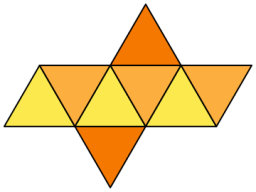Octahedron in a cube

The largest octahedron that can be placed inside a cubical box with sides equal to 72?

b =  88.1816

Step-by-step explanation:Did you find an error or inaccuracy? Feel free to write us. Thank you!Tips to related online calculators
Pythagorean theorem is the base for the right triangle calculator.

You need to know the following knowledge to solve this word math problem:

We encourage you to watch this tutorial video on this math problem: